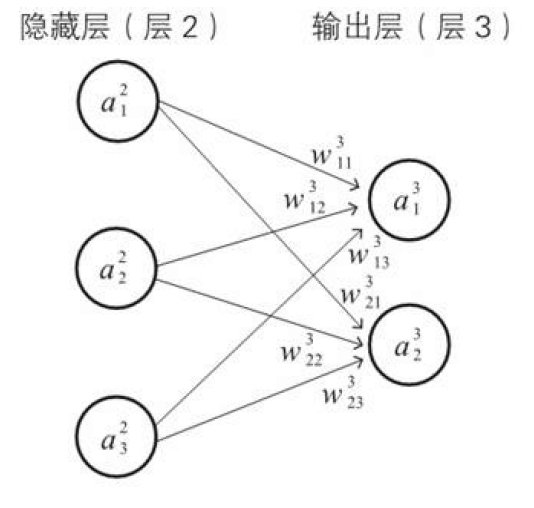# 深度学习数学基础(三)之简单数学

Posted by Naah on Saturday, Feb 08,2020 14:13:58

# 1 函数

## 1.2 二次函数

### 1.2.1 一元二次函数

1. 当$a>0$时，抛物线向上开口，向下凸起
2. 当$a<0$时，抛物线向下开口，向上凸起。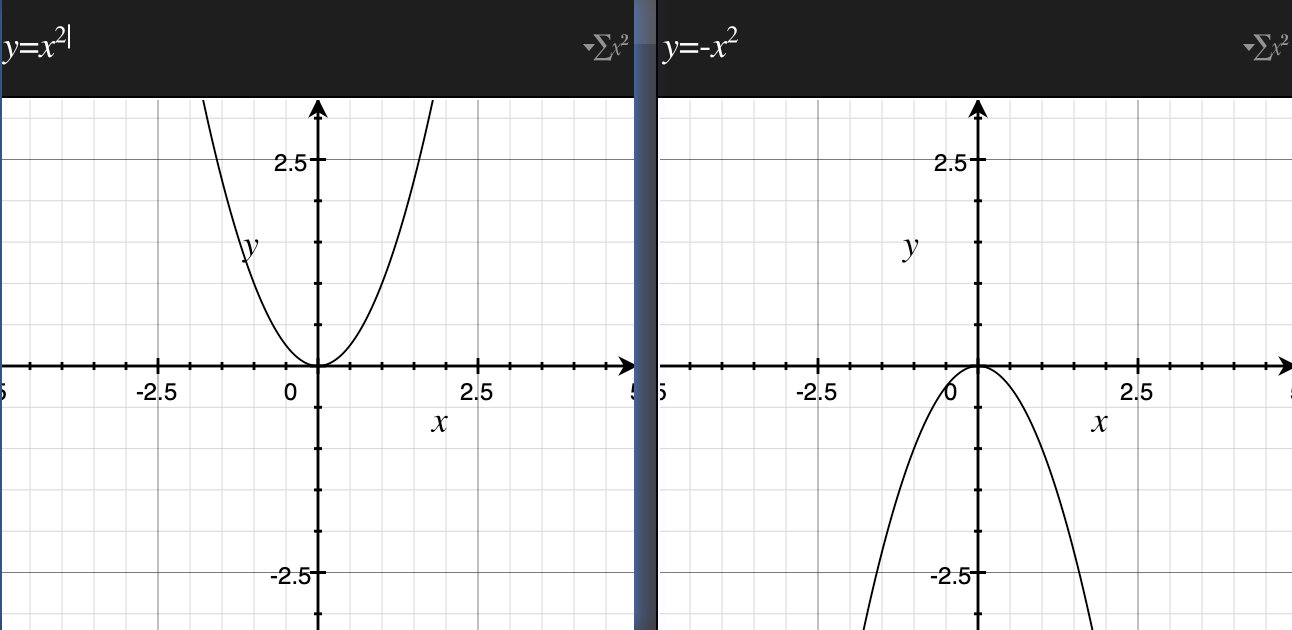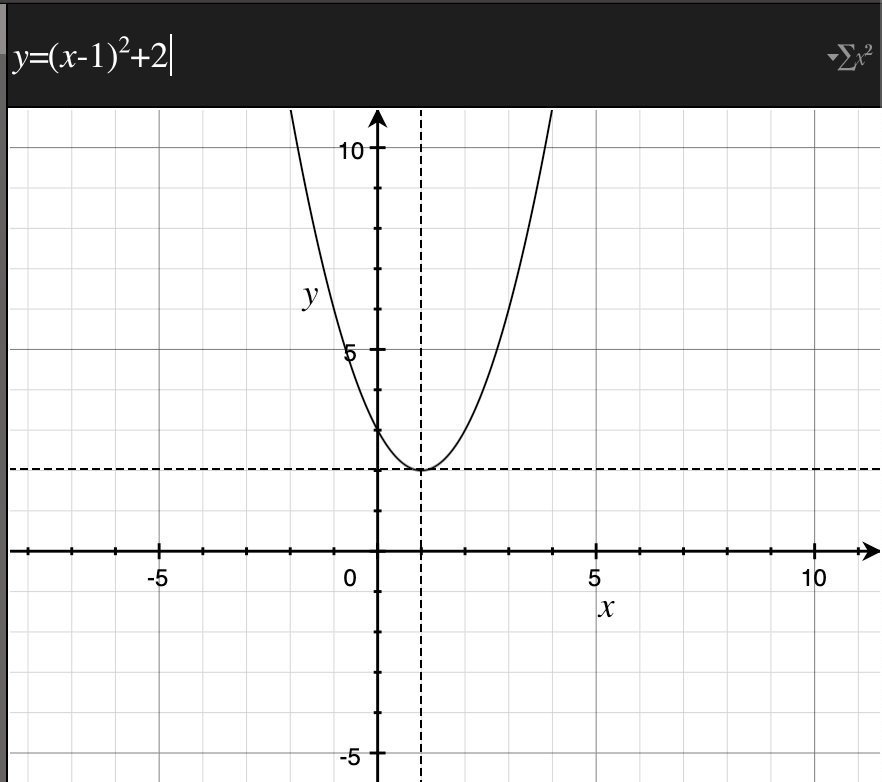### 1.2.2 多元二次函数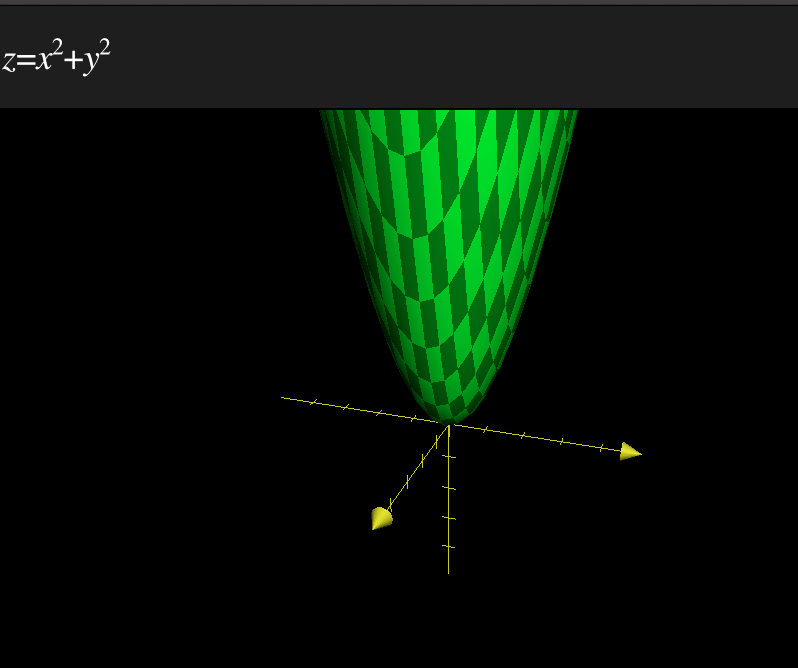## 1.3 单位阶跃函数

$$u(x)=\left\{ \begin{matrix} 0\quad (x<0) \\ 1\quad (x\ge 0) \end{matrix} \right\}$$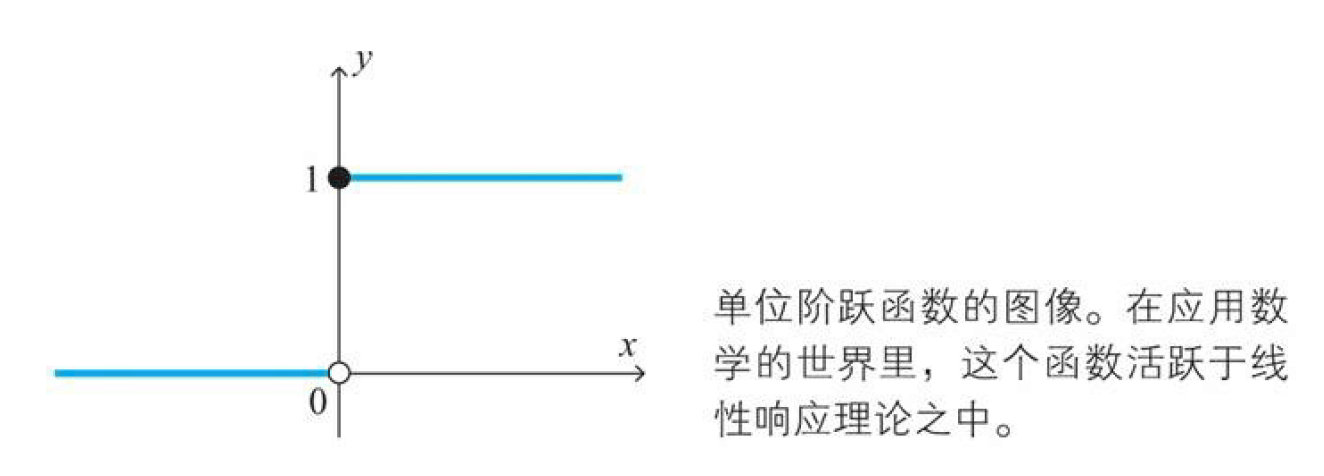## 1.5 正态分布的概率密度函数

1. 常数$\mu$：期望值（平均值）
2. $\sigma$：标注差

# 2 数列

## 2.1 数列的含义

数列就是数的序列，比如下面就是偶数列的数列 $$2,4,6,8,…$$

## 2.2 数列的通项公式

1. 加权输入：第$l$层的第$j$个神经元的加权输入用$z_j^l$
2. 输出：第$l$层的第$j$个神经元的输出用$a_j^l$

## 2.3 数列与递推关系式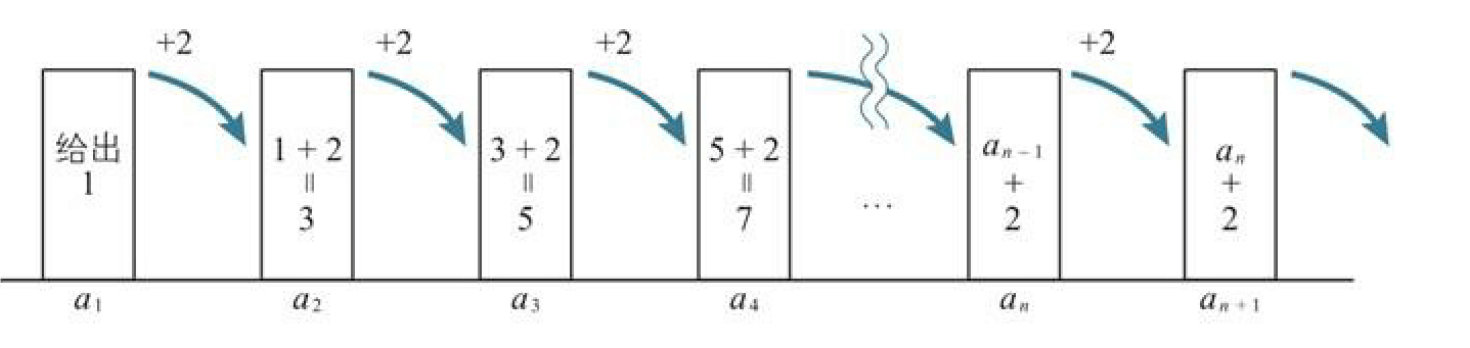## 2.4 联立递推关系式